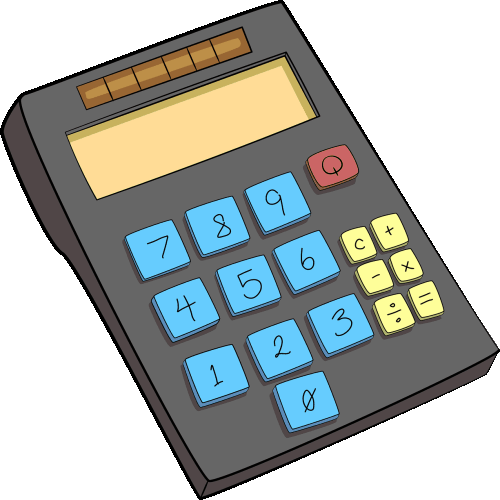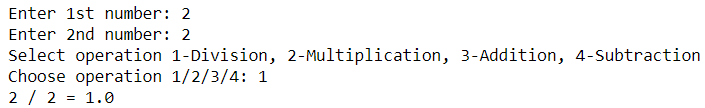# How To Make A Simple Python 3 Calculator Using FunctionsHere, you will learn how to make a simple Python 3 calculator. To understand this example, you should have the basic knowledge of Python programming concepts such as variables, operators, functions, and if-else statements.

## Steps To Make A Simple Calculator Using Python 3

Step 1: First, we will take two numbers input from the user using the input function.

Step 2: After that, we will create functions to perform arithmetic operations such as division, multiplication, subtraction, and addition. These functions will take two inputs (two numbers) and return the result of that operation.

To perform these operations, we will define four functions – multiplication(num1, num2), addition(num1, num2), subtraction(num1, num2), and divide(num1, num2).

Step 3: Next, we will provide the option to choose an operation (division, multiplication, subtraction, and addition). We can simply implement it by asking users to enter a digit to select the arithmetic operation they want to perform on the input numbers.

Users will see the following message on their console application – “Select operation 1-Division, 2-Multiplication, 3-Addition, 4-Subtraction.”

Step 4: Now, users can then choose the operation that they want to perform. Let’s suppose the user selects the first operation, then they will have to enter 1, and then our program will display the result of the operation.

### Python 3 code for a simple calculator

```#Python Program to Make a Simple Calculator

def multiplication(num1, num2):
return num1 * num2

return num1 + num2

def subtraction(num1, num2):
return num1 - num2

def divide(num1, num2):
return num1 / num2

value1 = int(input("Enter 1st number: "))
value2 = int(input("Enter 2nd number: "))

print("Select operation 1-Division, 2-Multiplication, 3-Addition, 4-Subtraction")
operation = int(input("Choose operation 1/2/3/4: "))

if operation == 1:
print(value1, "/", value2, "=", divide(value1, value2))

elif operation == 2:
print(value1, "*", value2, "=", multiplication(value1, value2))

elif operation == 3:
print(value1, "+", value2, "=", addition(value1, value2))
elif operation == 4:
print(value1, "-", value2, "=", subtraction(value1, value2))
else:
print("Enter correct operation")
```

Output:

Enter 1st Number: 2
Enter 2nd Number: 2
Select operation 1-Division, 2-Multiplication, 3-Addition, 4-Subtraction
Choose operation 1/2/3/4: 1
2 / 2 = 1.0Try it yourself:

### Summing Up

With this tutorial, you can create a basic Python 3 calculator. However, feel free to play with the code by adding more functionalities. Stay tuned, and we will also share the tutorial to build a GUI calculator application using Python.

In case, you have any queries, then do let me know in the comments section and I will try my best to assist you.

Scroll to Top